# 故宮下雪了！我花了45秒，用Python給它畫了一組手繪圖

（給

Python開發者

13日早晨，當北京市民拉開窗帘時發現，窗外雪花紛紛揚揚在空中飄落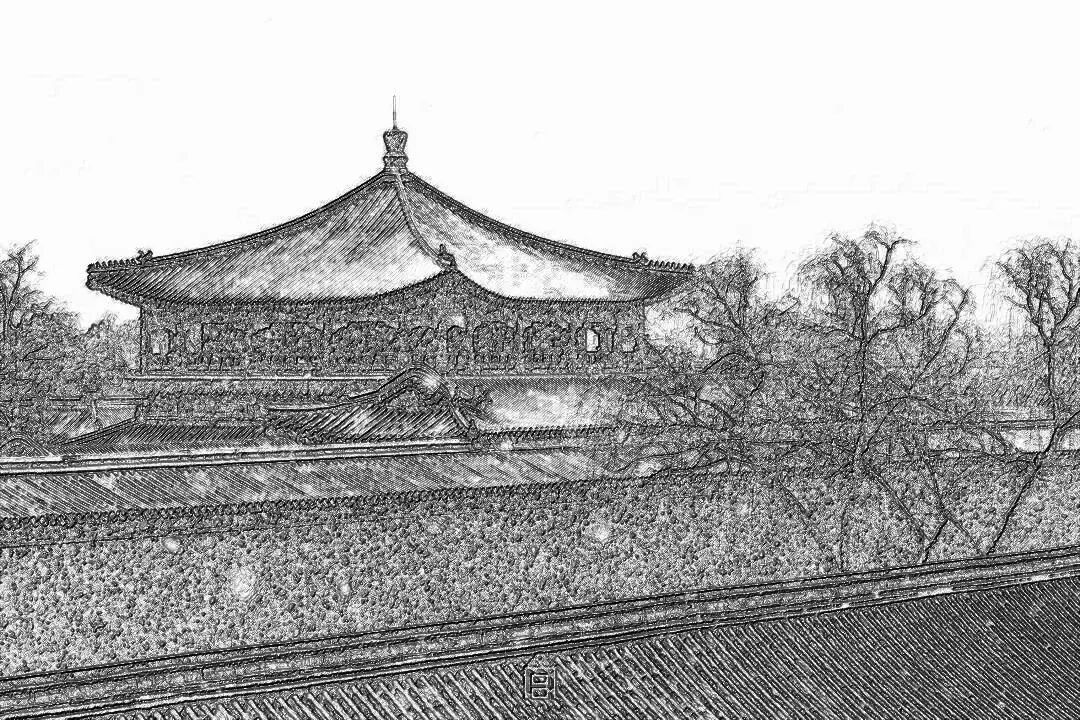• 黑白灰色；邊界線條較重；相同或相近色彩趨於白色；略有光源效果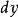，然後計算梯度角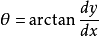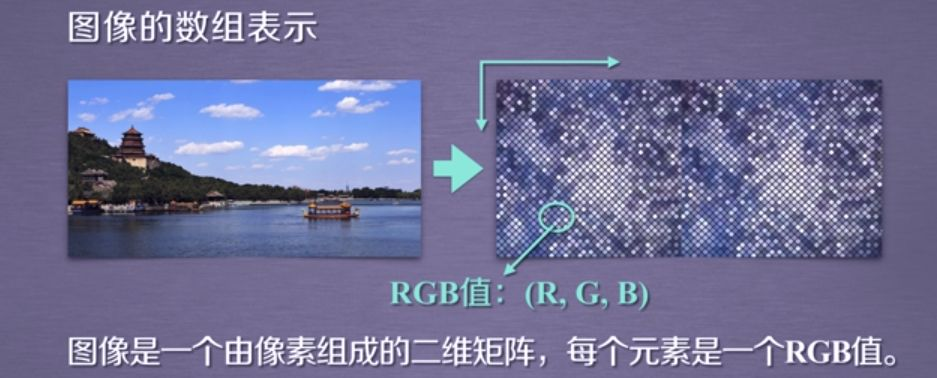• Image.open( ): 打開圖片

• np.array( ) : 將圖像轉化為數組

• convert("L"): 將圖片轉換成二維灰度圖片

• Image.fromarray( ): 將數組還原成圖像uint8格式

`from`

``` PIL import Imageimport numpy as np im = Image.open(r"C:UsersAdministratorDesktopgugong微信圖片_20190216152248.jpg" (adsbygoogle = window.adsbygoogle || []).push({}); ).convert("L")a=np.asarray(im).astype("float")print(a.shape,a.dtype)(1080, 608) float64#(1080, 608)分別表示高度，寬度```

1、梯度的重構

numpy的梯度函數的介紹

`grad=np.gradient(a)grad_x,grad_y=gradgrad_x = grad_x * depth / 100.`

`#對grad_x值進行歸一化`

`grad_y = grad_y * depth / 100.#對grad_y值進行歸一化`

2、構造guan光源效果np.cos(evc.el) : 單位光線在地平面上的投射長度

dx,dy,dz ：光源對x，y，z三方向的影響程度

3、梯度歸一化

• 構造x和y軸梯度的三維歸一化單位坐標系；

• 梯度與光源相互作用，將梯度轉化為灰度。

4、圖像生成

`from`

``` PIL import Imageimport numpy as npimport osimport joinimport time def image(sta,end,depths=10):    a = np.asarray(Image.open(sta).convert("L")).astype("float")    depth = depths  # 深度的取值範圍(0-100)，標準取10    grad = np.gradient(a)  # 取圖像灰度的梯度值    grad_x, grad_y = grad  # 分別取橫縱圖像梯度值    grad_x = grad_x * depth / 100.#對grad_x值進行歸一化    grad_y = grad_y * depth / 100.#對grad_y值進行歸一化    A = np.sqrt(grad_x ** 2 + grad_y ** 2 + 1.)    uni_x = grad_x / A    uni_y = grad_y / A    uni_z = 1. / A    vec_el = np.pi / 2.2  # 光源的俯視角度，弧度值    vec_az = np.pi / 4.  # 光源的方位角度，弧度值    dx = np.cos(vec_el) * np.cos(vec_az)  # 光源對x 軸的影響    dy = np.cos(vec_el) * np.sin(vec_az)  # 光源對y 軸的影響    dz = np.sin(vec_el)  # 光源對z 軸的影響    b = 255 * (dx * uni_x + dy * uni_y + dz * uni_z)  # 光源歸一化    b = b.clip(0, 255)    im = Image.fromarray(b.astype("uint8"))  # 重構圖像    im.save(end) def main():    xs=10    start_time = time.clock()    startss = os.listdir(r"C:UsersAdministratorDesktopgugong")    time.sleep(2)    for starts in startss:        start = "".join(starts)        sta = "C:/Users/Administrator/Desktop/gugong/" + start        end = "C:/Users/Administrator/Desktop/gugong/" + "HD_" + start        image(sta=sta,end=end,depths=xs)     end_time = time.clock()    print("程序運行了  ----" + str(end_time - start_time) + "   秒")    time.sleep(3) main()程序運行了  ----43.01828205879955   秒  #一共35張圖片```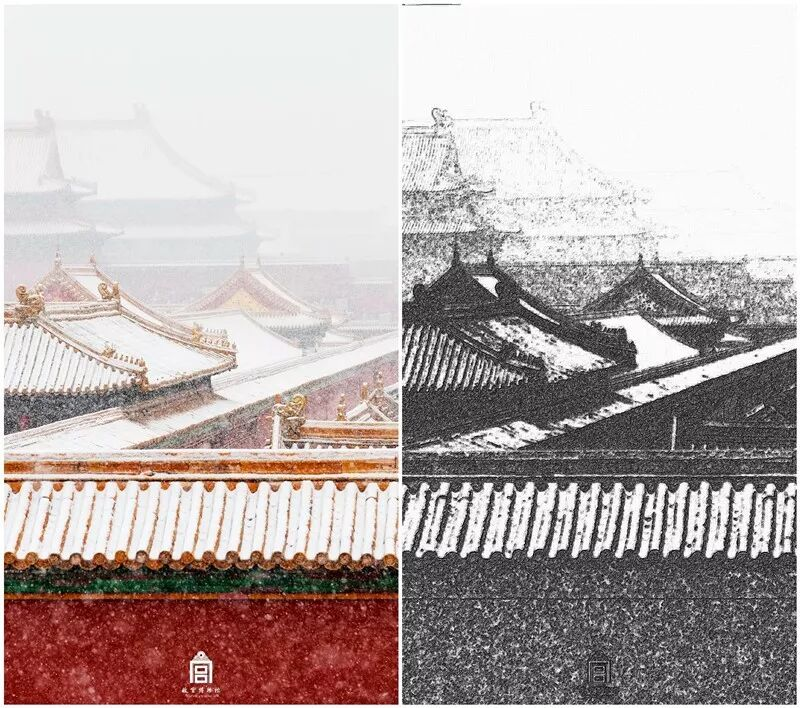http://www.icourse163.org/learn/BIT-1001870002?tid=1001963001#/learn/announce

【本文作者】

一名痴戀於 Python 的碼農，個人公號：「戀習Python」，在這裡我們一起用Python 做些有意義的事。

（點擊標題可跳轉閱讀）TAG:Python開發者 |